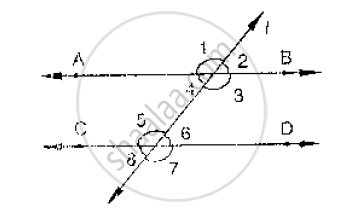# In Below Fig, Ab Cd and ∠1 and ∠2 Are in the Ratio 3 : 2. Determine All Angles from 1 to 8. - Mathematics

In below fig, AB CD and ∠1 and ∠2 are in the ratio 3 : 2. Determine all angles from 1 to 8.#### Solution

Let ∠1 = 3x and ∠2 = 2x

∠1and ∠2 are linear pair of angle

Now,  ∠1+ ∠2 = 180°

⇒ 3x + 2x = 180°

⇒ 5x = 180°

x = (180°)/5

⇒ x = 36°

∴∠1 = 3x = 108°, ∠2 = 2x = 72°

Vertically opposite angles are equal

∠1 = ∠3 = 108°

∠2 = ∠4 = 72°

∠6 = ∠7 = 108°

∠5 = ∠8 = 72°

Corresponding angles

∠1 = ∠5 = 108°

∠2 = ∠6 = 72°

Concept: Pairs of Angles
Is there an error in this question or solution?

#### APPEARS IN

RD Sharma Mathematics for Class 9
Chapter 10 Lines and Angles
Exercise 10.4 | Q 1 | Page 46